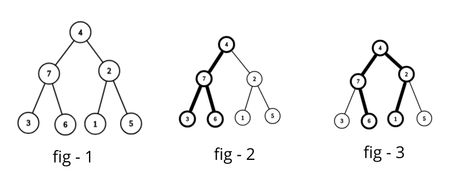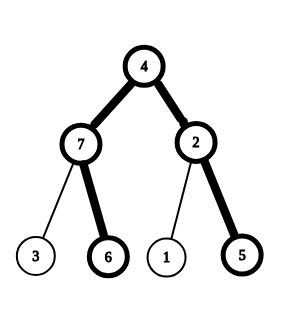# Afifa's ITSkfoozminusReplay of IUT 10th ICT Fe...
Limits 1s, 512 MB

Imagine some made up story here including Afifa (my little sister) and an Intelligent Transport System, which ultimately leads to the main problem.

You are given a connected tree containing $N$ nodes and $N - 1$ bidirectional edges. That means, you can go from one node to any other node. Every node $i$ has some weight $w_i$. Let's keep it simple and say that $w_i = i$ for the $i$-th node. Let this tree be called A.

Let's call a node terminal if it has one edge attached to it or no edge at all.

You have to pick a "part" of this tree that's also a tree. You can pick the entire tree as well. Let this new tree be called B.Fig - 1 denotes an example of tree $A$. Fig - 2 and fig - 3 shows two of the many possible ways to pick tree $B$. Now, you have to do this in a way so that the cost is maximum.

The cost of choosing tree $B = \text{(minimum weight among the terminals of B)} \times \text{(number of edges in B)}$.

For fig -2, the cost is $minimum(3, 4, 6) \times 3 = 9$ and for fig - 3, the cost is $minimum(1, 6) \times 4 = 4$. Neither of the costs are maximum.

## Input

The first line contains a single integer $N$ ($1 ≤ N ≤ 100000$) - number of nodes in the tree. Each of the next $N - 1$ lines contains two integers $u$ and $v$ ($1 ≤ u, v ≤ N$) - denoting an edge of the tree.

## Output

Print the maximum possible cost of new tree $B$.

## Sample

InputOutput
7
2 4
1 2
2 5
3 7
4 7
6 7

20Here, tree $B$, marked with bold edges, has two terminals with weight 5 and 6. Number of edges in $B$ equals 4.

So the cost is $minimum(5, 6) \times 4 = 20$, which is the maximum possible cost for this case.

### Submit